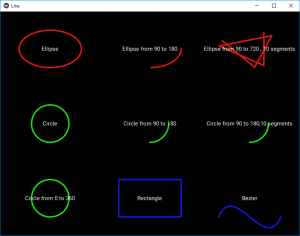# Python | Line (Canvas) in kivy

Kivy is a platform independent GUI tool in Python. As it can be run on Android, IOS, linux and Windows etc. It is basically used to develop the Android application, but it does not mean that it can not be used on Desktops applications.

## Line canvas:

Line is a vertex canvas instruction. It allows drawing of lines through points. This code shows how to use/draw the extended line drawing such as circles, ellipses, rectangles and Bezier and so on.

```Basic Approach:

1) import kivy
2) import kivy App
3) import Gridlayout
4) import widget
5) set minimum version(optional)
6) Create as much as widget class as needed
7) create the App class
8) return the widget/layout etc class
9) Run an instance of the class```

Implementation of Approach:

# Main.py

 `# kivy Lines Demo ` ` `  `# import kivy module   ` `import` `kivy   ` `     `  `# base Class of your App inherits from the App class.     ` `# app:always refers to the instance of your application     ` `from` `kivy.app ``import` `App   ` `     `  ` `  `# The GridLayout arranges children in a matrix.  ` `# It takes the available space and  ` `# divides it into columns and rows,  ` `# then adds widgets to the resulting “cells”.  ` `from` `kivy.uix.gridlayout ``import` `GridLayout  ` ` `  `# Widgets are elements of ` `# a graphical user interface that ` `# form part of the User Experience. ` `from` `kivy.uix.widget ``import` `Widget ` ` `  ` `  `############################################## ` `# Classes form Different types of line as  widgets ` ` `  `class` `LineEllipse1(Widget): ` `    ``pass` ` `  `class` `LineEllipse2(Widget): ` `    ``pass` ` `  `class` `LineEllipse3(Widget): ` `    ``pass` ` `  `class` `LineCircle1(Widget): ` `    ``pass` ` `  `class` `LineCircle2(Widget): ` `    ``pass` ` `  `class` `LineCircle3(Widget): ` `    ``pass` ` `  `class` `LineCircle4(Widget): ` `    ``pass` ` `  `class` `LineRectangle(Widget): ` `    ``pass` ` `  `class` `LineBezier(Widget): ` `    ``pass` ` `  ` `  `# Create the App class ` `class` `LineApp(App): ` `    ``def` `build(``self``): ` ` `  `        ``# Assign the number of coloumn, spacing and padding  ` `        ``root ``=` `GridLayout(cols ``=` `3``, padding ``=` `50``, spacing ``=` `100``) ` ` `  `        ``# Adding the widgets ` `        ``root.add_widget(LineEllipse1()) ` `        ``root.add_widget(LineEllipse2()) ` `        ``root.add_widget(LineEllipse3()) ` `        ``root.add_widget(LineCircle1()) ` `        ``root.add_widget(LineCircle2()) ` `        ``root.add_widget(LineCircle3()) ` `        ``root.add_widget(LineCircle4()) ` `        ``root.add_widget(LineRectangle()) ` `        ``root.add_widget(LineBezier()) ` `        ``return` `root ` ` `  `# Run the App class ` `if` `__name__ ``=``=` `'__main__'``: ` `    ``LineApp().run() `

Line.kv file:

 `# Line.kv file of the code ` ` `  `# Creating Different types of Lines(or shapes through line) ` ` `  `########################################### ` ` `  `# Row 1: ` ` `  `# Ellipse(1st row 1st element) ` ` `  `: ` ` `  `    ``# Creating Canvas ` `    ``canvas: ` `        ``Color: ` `            ``rgba: ``1``, .``1``, .``1``, .``9` `        ``# Ellipse Creation ` `        ``Line: ` `            ``width: ``2.` `            ``ellipse: (``self``.x, ``self``.y, ``self``.width, ``self``.height) ` ` `  `    ``# Label the figure  ` `    ``Label: ` `        ``center: root.center ` `        ``text: ``'Ellipse'` ` `  ` `  `############################################ ` ` `  `# Ellipse from 90 to 180((1st row 2nd element)) ` ` `  `: ` `    ``canvas: ` `        ``Color: ` `            ``rgba: ``1``, .``1``, .``1``, .``9` `        ``Line: ` `            ``width: ``2.` `            ``ellipse: (``self``.x, ``self``.y, ``self``.width, ``self``.height, ``90``, ``180``) ` `    ``Label: ` `        ``center: root.center ` `        ``text: ``'Ellipse from 90 to 180'` ` `  ` `  `############################################ ` ` `  ` `  `# Ellipse from 90 to 720, 10 segments(1st row 3rd element) ` ` `  `: ` `    ``canvas: ` `        ``Color: ` `            ``rgba: ``1``, .``1``, .``1``, .``9` `        ``Line: ` `            ``width: ``2.` `            ``ellipse: (``self``.x, ``self``.y, ``self``.width, ``self``.height, ``90``, ``720``, ``10``) ` `    ``Label: ` `        ``center: root.center ` `        ``text: ``'Ellipse from 90 to 720, 10 segments'` `        ``halign: ``'center'` ` `  `############################################ ` ` `  `# Circle(2nd row 1st element) ` `: ` `    ``canvas: ` `        ``Color: ` `            ``rgba: .``1``, ``1``, .``1``, .``9` `        ``Line: ` `            ``width: ``2.` `            ``circle: ` `                ``(``self``.center_x, ``self``.center_y, ``min``(``self``.width, ``self``.height) ` `                ``/` `2``) ` `    ``Label: ` `        ``center: root.center ` `        ``text: ``'Circle'` ` `  `############################################ ` ` `  `# Circle from 90 to 180(2nd row 2nd element) ` `: ` `    ``canvas: ` `        ``Color: ` `            ``rgba: .``1``, ``1``, .``1``, .``9` `        ``Line: ` `            ``width: ``2.` `            ``circle: ` `                ``(``self``.center_x, ``self``.center_y, ``min``(``self``.width, ``self``.height) ` `                ``/` `2``, ``90``, ``180``) ` `    ``Label: ` `        ``center: root.center ` `        ``text: ``'Circle from 90 to 180'` ` `  `############################################ ` `# Circle from 90 to 180, 10 segments(1st row 3rd element) ` `: ` `    ``canvas: ` `        ``Color: ` `            ``rgba: .``1``, ``1``, .``1``, .``9` `        ``Line: ` `            ``width: ``2.` `            ``circle: ` `                ``(``self``.center_x, ``self``.center_y, ``min``(``self``.width, ``self``.height) ` `                ``/` `2``, ``90``, ``180``, ``10``) ` `    ``Label: ` `        ``center: root.center ` `        ``text: ``'Circle from 90 to 180, 10 segments'` `        ``halign: ``'center'` ` `  `############################################ ` ` `  `# Circle from 0 to 360 (3rd row 1st element) ` `: ` `    ``canvas: ` `        ``Color: ` `            ``rgba: .``1``, ``1``, .``1``, .``9` `        ``Line: ` `            ``width: ``2.` `            ``circle: ` `                ``(``self``.center_x, ``self``.center_y, ``min``(``self``.width, ``self``.height) ` `                ``/` `2``, ``0``, ``360``) ` `    ``Label: ` `        ``center: root.center ` `        ``text: ``'Circle from 0 to 360'` `        ``halign: ``'center'` ` `  `############################################ ` ` `  `# Rectangle (3rd row 2nd element) ` `: ` `    ``canvas: ` `        ``Color: ` `            ``rgba: .``1``, .``1``, ``1``, .``9` `        ``Line: ` `            ``width: ``2.` `            ``rectangle: (``self``.x, ``self``.y, ``self``.width, ``self``.height) ` `    ``Label: ` `        ``center: root.center ` `        ``text: ``'Rectangle'` ` `  `############################################ ` ` `  `# Bezier (3rd row 3rd element) ` `: ` `    ``canvas: ` `        ``Color: ` `            ``rgba: .``1``, .``1``, ``1``, .``9` `        ``Line: ` `            ``width: ``2.` `            ``bezier: ` `                ``(``self``.x, ``self``.y, ``self``.center_x ``-` `40``, ``self``.y ``+` `100``, ` `                ``self``.center_x ``+` `40``, ``self``.y ``-` `100``, ``self``.right, ``self``.y) ` `    ``Label: ` `        ``center: root.center ` `        ``text: ``'Bezier'`

Output:Attention geek! Strengthen your foundations with the Python Programming Foundation Course and learn the basics.

To begin with, your interview preparations Enhance your Data Structures concepts with the Python DS Course.

My Personal Notes arrow_drop_upCompetative Programmer

If you like GeeksforGeeks and would like to contribute, you can also write an article using contribute.geeksforgeeks.org or mail your article to contribute@geeksforgeeks.org. See your article appearing on the GeeksforGeeks main page and help other Geeks.

Please Improve this article if you find anything incorrect by clicking on the "Improve Article" button below.

Article Tags :

Be the First to upvote.

Please write to us at contribute@geeksforgeeks.org to report any issue with the above content.